# RS Aggarwal Class 7 Solutions Chapter 7 Linear Equations in One Variable Ex 7A

In this chapter, we provide RS Aggarwal Solutions for Class 7 Chapter 7 Linear Equations in One Variable Ex 7A for English medium students, Which will very helpful for every student in their exams. Students can download the latest RS Aggarwal Solutions for Class 7 Chapter 7 Linear Equations in One Variable Ex 7A Maths pdf, free RS Aggarwal Solutions Class 7 Chapter 7 Linear Equations in One Variable Ex 7A Maths book pdf download. Now you will get step by step solution to each question.

### RS Aggarwal Solutions for Class 7 Chapter 7 Linear Equations in One Variable Ex 7A Download PDF

Question 1.
Solution:
3x – 5 = 0
3x – 5 + 5 = 0 + 5
⇒ 3x = 5
⇒ x = 53
Check:
L.H.S. = 3x – 5
= 3 x 53 – 5
= 5 – 5
= 0
= R.H.S.
Hence x = 53

Question 2.
Solution:
8x – 3 = 9 – 2x
⇒ 8x + 2x = 9 + 3 (By transposing)
⇒ 10x = 12Question 3.
Solution:
7 – 5x = 5 – 7x
⇒ – 5x + 7x = 5 – 7 (By transposing)
⇒ 2x = -2
x = -1
Check:
L.H.S. = 7 – 5x = 7 – 5(-1) = 7 + 5 = 12
R.H.S. = 5 – 7x = 5 – 7(-1) = 5 + 7 = 12
L.H.S. = R.H.S.
Hence x = -1

Question 4.
Solution:
3 + 2x = 1 – x
⇒ 2x + x = 1 – 3 (By transposing)
⇒ 3x = -2Question 5.
Solution:
2(x – 2) + 3(4x – 1) = 0
⇒ 2x – 4 + 12x – 3 = 0
⇒ 2x + 12x = 4 + 3 (By transposing)
⇒ 14x = 7
⇒ x = 714 = 12
Check : L.H.S. = 2(x – 2) + 3 (4x -1)Question 6.
Solution:
5 (2x – 3) – 3(3x – 7) = 5
⇒ 10x – 15 – 9x + 21 = 5
⇒ 10x – 9x – 15 + 21 = 5
⇒ 10x – 9x = 5 + 15 – 21 (By transposing)
⇒ x = 20 – 21 = -1
⇒ x = -1
Check:
L.H.S. = 5 (2x – 3) – 3(3x – 7)
= 5[2 x (-1) -3] -3[3 (-1) -7] = 5[-2 – 3] – 3[-3 – 7]
= 5 x (-5) -3 x (-10)
= -25 + 30
= 5 = R.H.S.
Hence x = -1

Question 7.
Solution:Question 8.
Solution: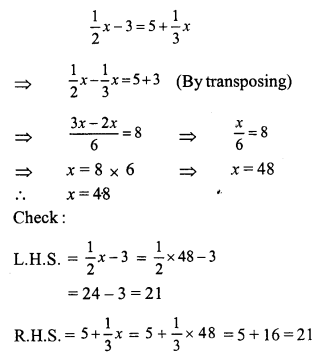L.H.S. = R.H.S.
Hence x = 48

Question 9.
Solution:Question 10.
Solution:
3x + 2(x + 2) = 20 – (2x – 5)
⇒ 3x + 2x + 4 = 20 – 2x + 5
⇒ 5x + 4 = 25 – 2x
⇒ 5x + 2x = 25 – 4 (By transposing)
⇒ 7x = 21
⇒ x = 3
Check:
L.H.S.= 3x + [2(x + 2)] = 3 x 3 + 2(3 + 2) = 9 + 2 x 5 = 9 + 10 = 19
R.H.S. = 20 – (2x – 5) = 20 – (2 x 3 – 5) = 20 – (6 – 5) = 20 – 1 = 19
L.H.S. = R.H.S.
Hence x = 3

Question 11.
Solution:
13(y – 4) – 3(y – 9) – 5(y + 4) = 0
⇒ 13y – 52 – 3y + 27 – 5y – 20 = 0
⇒ 13y – 3y – 5y – 52 + 27 – 20 = 0
⇒ 13y – 8y – 72 + 27 = 0
⇒ 5y – 45 = 0
⇒ 5y = 45 (By transposing)
⇒ y = 9
Check:
L.H.S. = 13(y – 4) – 3(y – 9) – 5(y + 4)
= 13(9 – 4) – 3(9 – 9) – 5(9 + 4)
= 13 x 5 – 3 x 0 – 5 x 13
= 65 – 0 – 65 = 0 = R.H.S.
Hence y = 9

Question 12.
Solution:
2m+53 = 3m – 10
⇒ 2m + 5 = 3 (3m – 10) (By cross multiplication)
⇒ 2m + 5 = 9m – 30
⇒ 2m – 9m = -30 – 5
⇒ -7m = -35
⇒ m = 5
m = 5
Check:R.H.S. = 3m – 10 = 3 x 5 – 10 = 15 – 10 = 5
L.H.S. = R.H.S.
Hence m = 5

Question 13.
Solution:
6(3x + 2) – 5(6x – 1) = 3(x – 8) – 5(7x – 6) + 9x
⇒ 18x + 12 – 30x + 5 = 3x – 24 – 35x + 30 + 9x
⇒ 18x – 30x + 12 + 5 = 3x – 35x + 9x – 24 + 30
⇒ -12x + 17 = -23x + 6
⇒ – 12x + 23x = 6 – 17
⇒ 11x = -11
x = – 1
Check:
L.H.S. = 6(3x + 2) – 5(6x – 1)
= 6[3x (-1) + 2] – 5[6 x (-1) x -1]
= 6[-3 + 2] – 5[-6 – 1]
= 6 x (-1) – 5 x (-7)
= -6 + 35 = 29
R.H.S. = 3(x – 8) – 5 (7x – 6) + 9x
= 3[-1 – 8] -5 [7 x (-1) – 6] + 9 (-1)
= 3 x (-9) – 5 [-7 – 6] – 9
= -27 – 5(-13) – 9
= -27 + 65 – 9
= 65 – 36 = 29 .
L.H.S. = R.H.S.
Hence x = -1

Question 14.
Solution:
t – (2t + 5) – 5(1 – 2t) = 2(3 + 4t) – 3(t – 4)
⇒ t – 2t – 5 – 5 + 10t = 6 + 8t – 3t + 12t
⇒ t – 2t + 10t – 10 = 8t – 3t + 18
⇒ 9t – 10 = 5t + 18
⇒ 9t – 5t = 18 + 10 (By transposing)
⇒ 4t = 28
⇒ t = 7
Check:
L.H.S. = t – [2t + 5] -5[1 – 2t]
= 7 – [2 x 7 + 5] – 5[1 – 2 x 7]
= 7 – [14 + 5] – 5 [1 – 14]
= 7 – 19 – 5(-13)
= 7 – 19 + 65
= 72 – 19 = 53
R.H.S. = 2[3 + 4t) – 3(t – 4)
= 2 (3 + 4 x 7) – 3(7 – 4)
= 2(3 + 28) – 3(3)
= 2(31) – 9 = 62 – 9 = 53
L.H.S. = R.H.S.
Hence t = 7 Ans.

Question 15.
Solution:Question 16.
Solution: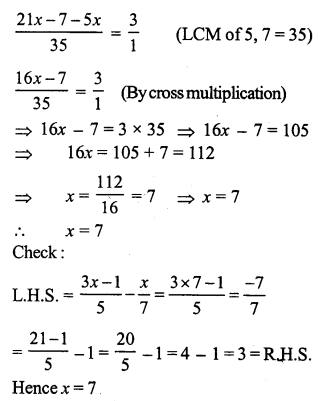Question 17.
Solution: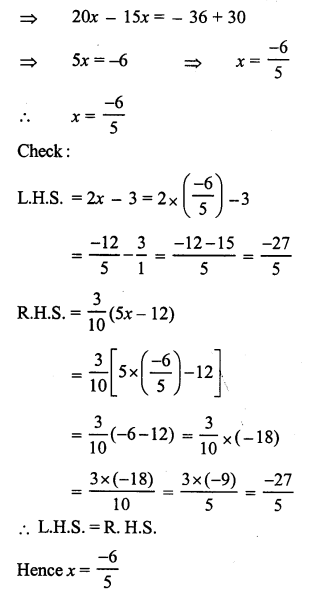Question 18.
Solution: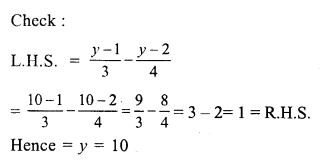Question 19.
Solution:Question 20.
Solution: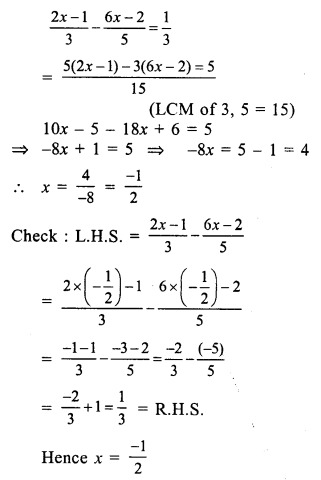Question 21.
Solution: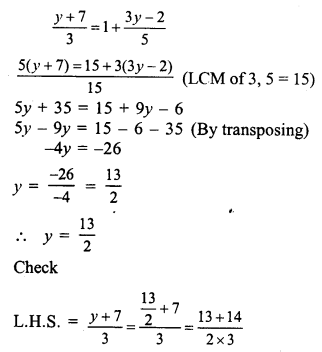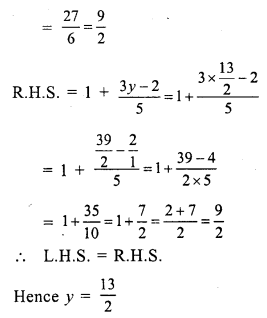Question 22.
Solution:Question 23.
Solution:Question 24.
Solution:Question 25.
Solution: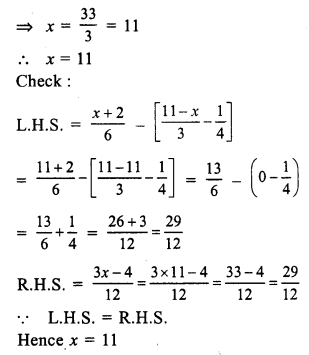Question 26.
Solution:Question 27.
Solution:Question 28.
Solution:
0.18 (5x – 4) = 0.5x + 0.8Question 29.
Solution:
2.4 (3 – x) – 0.6 (2x – 3) = 0
⇒ 7.2 – 2.4x – 1.2x + 1.8 = 0
⇒ -2.4x – 1.2x = – (7.2 + 1.8).
L.H.S. = 2.4 (3 – x) – 0.6 (2x – 3)
⇒ 2.4 (3 – 2.5) – 0.6 (2 x 2.5 – 3)
⇒ 2.4 (0.5) – 0.6 (5 – 3)
⇒ 1.2 – 0.6 x 2 = 1.2 – 1.2 = 0 = R.H.S.
Hence x = 2.5

Question 30.
Solution:
0.5x – (0.8 – 0.2x) = 0.2 – 0.3x
⇒ 0.5x – 0.8 + 0.2x = 0.2 – 0.3x
⇒ 0.5x + 0.2x + 0.3x = 0.2 + 0.8
⇒ 1.0x = 1.0
⇒ x = 1
Check :
L.H.S. = 0.5x – (0.8 – 0.2x)
= 0.5 x 1 – (0.8 – 0.2 x 1)
= 0.5 – (0.8 – 0.2) = 0.5 – 0.6 = -0.1
R.H.S. = 0.2 – 0.3x = 0.2 – 0.3 x 1 = 0.2 – 0.3 = -0.1
L.H.S. = R.H.S.
Hence x = 1

Question 31.
Solution: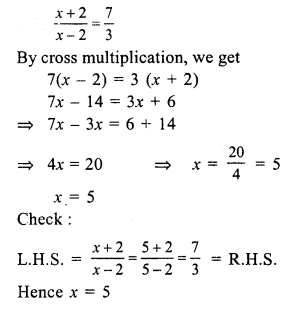Question 32.
Solution:All Chapter RS Aggarwal Solutions For Class 7 Maths

—————————————————————————–

All Subject NCERT Exemplar Problems Solutions For Class 7

All Subject NCERT Solutions For Class 7

*************************************************

I think you got complete solutions for this chapter. If You have any queries regarding this chapter, please comment on the below section our subject teacher will answer you. We tried our best to give complete solutions so you got good marks in your exam.

If these solutions have helped you, you can also share rsaggarwalsolutions.in to your friends.